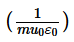>  Previous Year Questions (JEE Mains)

# Previous Year Questions (JEE Mains)

Test Description

## 11 Questions MCQ Test | Previous Year Questions (JEE Mains)

 1 Crore+ students have signed up on EduRev. Have you?
Previous Year Questions (JEE Mains) - Question 1

### Identify the pair whose dimensions are equal.                     [AIEEE 2002]

Previous Year Questions (JEE Mains) - Question 2

### The physical quantities not having same dimensions are                   [AIEEE 2003]

Previous Year Questions (JEE Mains) - Question 3

### Dimensions of, where symbols have their usual meaning, are                       [AIEEE 2003]

Previous Year Questions (JEE Mains) - Question 4

Which one of the following represents the correct dimensions of the coefficient of viscosity?     [AIEEE 2004]

Previous Year Questions (JEE Mains) - Question 5

Out of the following pairs, which one does not have identical dimensions ?            [AIEEE 2005]

Previous Year Questions (JEE Mains) - Question 6

The 'rad' is the correct unit used to report the measurement of                        [AIEEE 2006]

Previous Year Questions (JEE Mains) - Question 7

Which of the following units denotes the dimensions [ML2/Q2], where Q denotes the electric charges ? [AIEEE 2006]

Previous Year Questions (JEE Mains) - Question 8

A body of mass m = 3.513 kg is moving along the x-axis with a speed of 5.00 ms-1. The magnitude of its
momentum is recorded as             [AIEEE 2008]

Previous Year Questions (JEE Mains) - Question 9

The dimensions of magnetic field in M, L, T and C (coulomb) is given as          [AIEEE 2008]

Previous Year Questions (JEE Mains) - Question 10

In an experiment the angles are required to be measured using an instrument. 29 divisions of the main scale exactly coincide with the 30 divisions of the vernier scale. If the smallest division of the main scale is half-a-degree (= 0.5°), then the least count of the instrument is              [AIEEE 2009]

Previous Year Questions (JEE Mains) - Question 11

The respective number of significant figures for the numbers 23.023, 0.0003 and 21 × 10 -3 are

 Use Code STAYHOME200 and get INR 200 additional OFF Use Coupon Code